ExactNumberQ, InexactNumberQ, NumberQ, NumericQ

ExactNumberQ is undocumented, but as far as I can tell it returns True if it's argument has the head Integer, Rational, or if the argument is complex with infinite precision.  It seems ExactNumberQ returns False in every other case as demonstrated with the next input.  You may not be familiar with the feature Root that I use below, but it's well documented in the Help Browser and The Mathematica Book.  I suggest you refer to this documentation if you are confused with the use of Root below.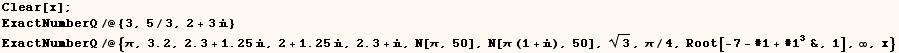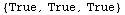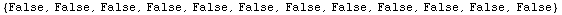InexactNumberQ is also undocumented it seems InexactNumberQ returns True if it's argument is an approximate number (real or complex), and returns False in every other case.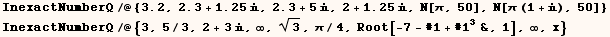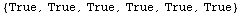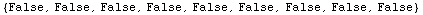NumberQ is documented and it's usage message is given below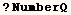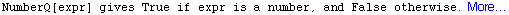It seems NumberQ  returns True whenever it's argument has the head Integer, Rational, Real, or Complex and False in every other case.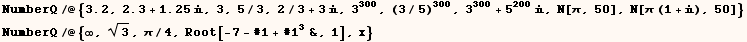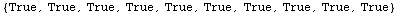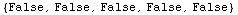NumericQ is also documented and it's usage message is given below.  NumericQ  recognizes things like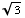as numeric values as demonstrated below.  Infinity and Indeterminate are not considered numeric.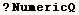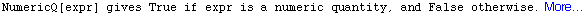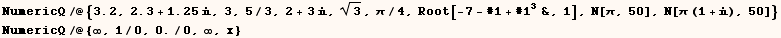Created by Mathematica  (May 16, 2004)

Back to Ted’s Tricks index page